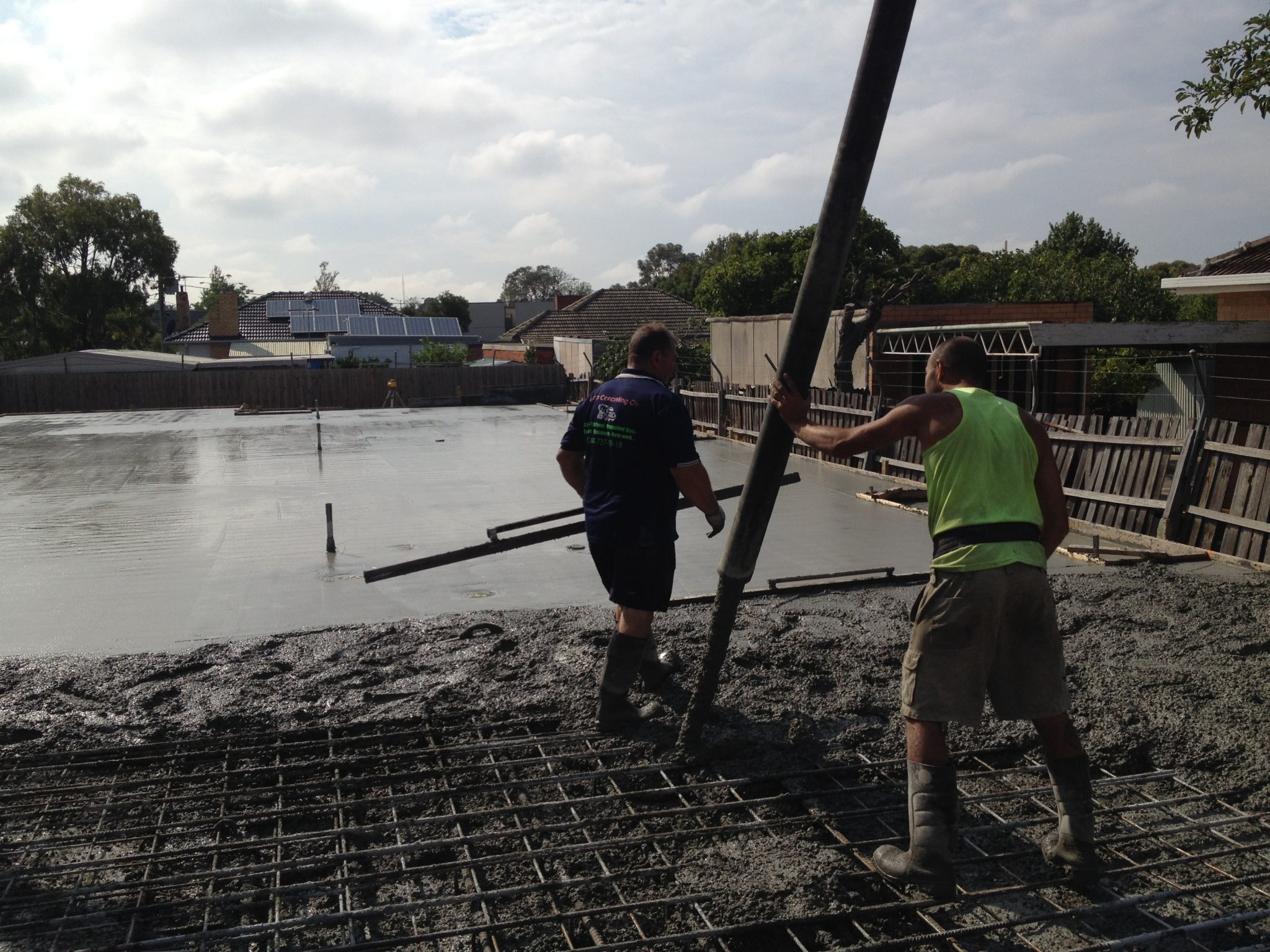Concrete Calculator

I want to enter my dimensions in

 1. Cubic Cylindrical L W D = 1. Cubic Cylindrical D H N = 2. Cubic Cylindrical L W D = 2. Cubic Cylindrical D H N = 3. Cubic Cylindrical L W D = 3. Cubic Cylindrical D H N = 4. Cubic Cylindrical L W D = 4. Cubic Cylindrical D H N = 5. Cubic Cylindrical L W D = 5. Cubic Cylindrical D H N = Total (rounded up to nearest 0.2m3) =

L:
Length
W:
Width
D:
Depth

#### Cylindrical

D:
Diameter
H:
Height
N:
Number of cylindersThis calculator is provided for your convenience only.

# Normal Class ConcreteDesigned for residential home projects, paving, footpaths, driveways and low rise applications. Normal class concrete to AS1379, compressive strengths of 20, 25, 32, 40 and 50MPa, utilising modern concrete additives to improve workability and finishing times.